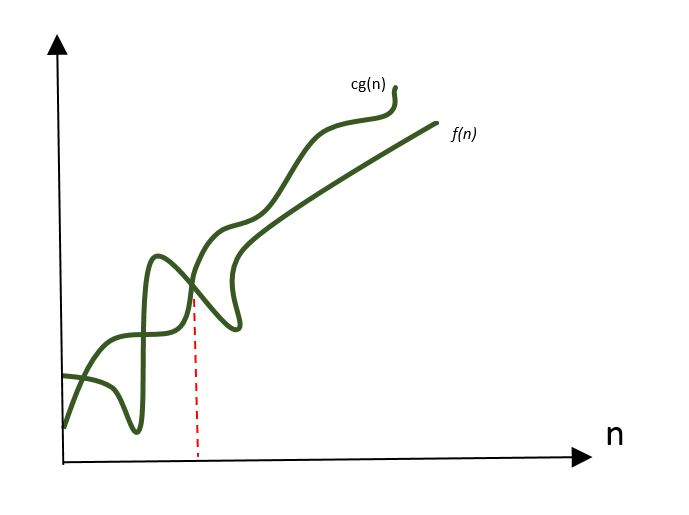# GATE | GATE-CS-2000 | Question 40

• Last Updated : 28 Jun, 2021

Consider the following functionsWhich of the following is true?
(A) h(n) is O(f(n))

Attention reader! Don’t stop learning now.  Practice GATE exam well before the actual exam with the subject-wise and overall quizzes available in GATE Test Series Course.

Learn all GATE CS concepts with Free Live Classes on our youtube channel.

(B) h(n) is O(g(n))
(C) g(n) is not O(f(n))
(D) f(n) is O(g(n))

Explanation: Big-oh notation:

Let f and g be two functions defined on real number. One writes f(n) = O(g(n)) if there is a positive constant M such that for all sufficiently large values of n, the absolute value of f(n) is at most M multiplied by the absolute value of g(n). That is, f(n) = O(g(n)) if and only if there exists a positive real number M and a real number n0 such that f(n)≤M(g(n)), for all n≥n0.In Big-oh notation, we only make comparison between two functions by considering larger values of n. To solve question like this we can take larger value of n and then compare the values of different function.

f(n) = 3(n^32)=3*(2^10)^32=3*2^320

g(n) = 2^320

h(n)=1024!

So relation between the functions can be:

1.f(n) and g(n) are of same order, so f(n) is O(g(n)) and g(n)=O(f(n)). Hence option C is wrong.
2.h(n) is n! Which is of higher order than f(n) and g(n). So options A and B are wrong.

This solution is contributed by Nirmal Bharadwaj

Quiz of this Question

My Personal Notes arrow_drop_up